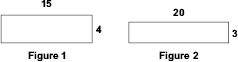# User ForumSubject :IMO    Class : Class 5Which statement about the given figures is false?

A Area of figure 2 is more than area of figure 1
B Perimeter of figure 2 is more than perimeter of figure 1
C Length of figure 1 is less than the length of figure 2
D None of these

How to do it?

## Ans 1:

Class : Class 10
vayam guptaexcelsior american schoolclass: 5IT IS WRONG ,ANSWER [ C ]

## Ans 2:

Class : Class 8
area of figure 1 = L*B=15*4=60 area of figure 2= L*B=20*3=60 so both are same ... this is the only false statement